Jet Set Go! All about Aeroplanes Jet Set Go! All about Aeroplanes

# Newton's Laws of Motion

While Newton’s laws of motion may seem obvious to us today, they were considered revolutionary centuries ago. The three laws of motion help us understand how objects behave when standing still, when moving and when forces act upon them. This article describes Sir Newton’s three laws and a summary of what they mean.

## Newton’s First Law of Motion

Newton’s first law of motion implies that things cannot start, stop, or change direction all by themselves, and it requires some force from the outside to cause such a change. This property of massive bodies to resist changes in their state of motion is called inertia. The first law of motion is also known as the law of inertia.

Newton’s 1st law states that a body at rest or uniform motion will continue to be at rest or uniform motion until and unless a net external force acts on it.

The crucial point here is that if there is no net force resulting from unbalanced forces acting on an object, the object will maintain a constant velocity. If that velocity is zero, then the object remains at rest. And if an additional external force is applied, the velocity will change because of the force.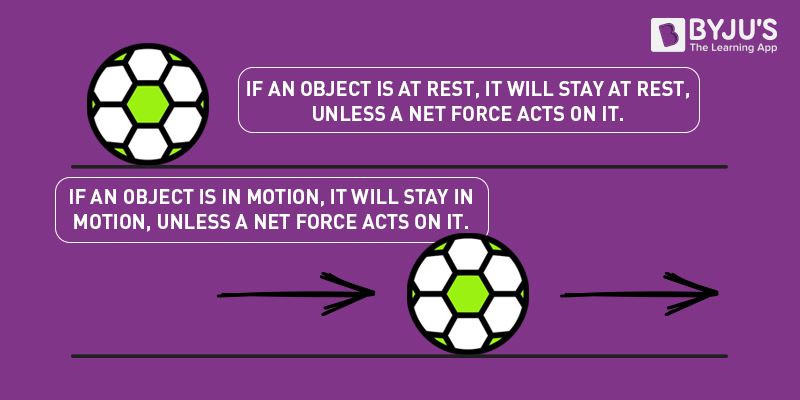Read More: Newton’s First Law of Motion

## Newton’s Second Law of Motion

Newton’s second law of motion describes what happens to the massive body when acted upon by an external force. The second law of motion states that the force acting on the body is equal to the product of its mass and acceleration.

Newton’s 2nd law states that the acceleration of an object as produced by a net force is directly proportional to the magnitude of the net force, in the same direction as the net force, and inversely proportional to the object’s mass.

Newton’s second law describes precisely how much an object will accelerate for a given net force.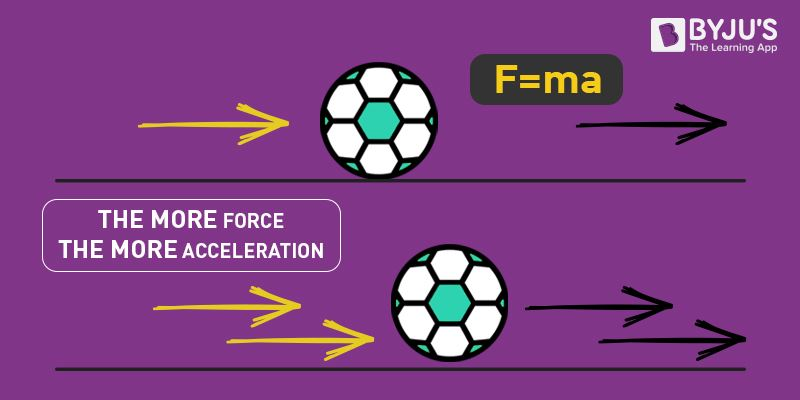Mathematically, we express the second law of motion as follows:

$$\begin{array}{l}f\propto \frac{dP}{dt}\\ \\ \Rightarrow f\propto \frac{mv-mu}{t}\\ \\ \Rightarrow f\propto\frac{m(v-u)}{t}\\ \\ \Rightarrow f\: \propto \: ma\\ \\ \Rightarrow f=k\, ma\end{array}$$
Where f is force, P is linear momentum, m is mass of the object, u is initial velocity, v is final velocity and a is acceleration.

In the equation, k is the constant of proportionality, and it is equal to 1 when the values are taken in the SI unit. Hence, the final expression will be,

$$\begin{array}{l}F=ma\end{array}$$

Read More: Newton’s Second Law of Motion

## Newton’s Third Law of Motion

Newton’s third law of motion describes what happens to the body when it exerts a force on another body.

Newton’s 3rd law states that there is an equal and opposite reaction for every action.

When two bodies interact, they apply forces on each other that are equal in magnitude and opposite in direction. To understand Newton’s third law with the help of an example, let us consider a book resting on a table. The book applies a downward force equal to its weight on the table. According to the third law of motion, the table applies an equal and opposite force to the book. This force occurs because the book slightly deforms the table; as a result, the table pushes back on the book like a coiled spring. Newton’s third law of motion implies the conservation of momentum.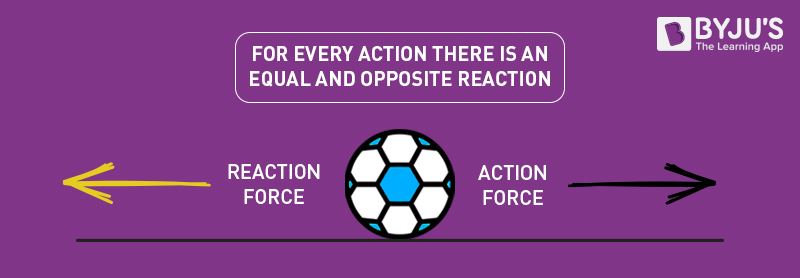Read More: Newton’s Third Law of Motion

## Laws of Motion Questions

1) While driving to work, Naveen keeps his wallet in the passenger seat, and by the time he gets to work, his wallet would have fallen on the floor in front of the passenger seat. One day, he asks you to explain why this happens in physics. What do you say?

We can explain this to Naveen in terms of Newton’s first law of motion. The wallet, due to inertia, travels forward with the car until acted upon by another force, in this case, the force of the car floor.

2) Which of Newton’s laws best explains how a magician can pull a tablecloth from underneath dishes?

Newton’s first law of motion can explain how a magician pulls a tablecloth underneath the dishes. A negligible horizontal force is applied during the process. As per Newton’s first law of motion, the dishes and glasses remain in their state of motion (rest); as a result, they remain undisturbed. The table cloth in the trick is made so slippery that it doesn’t apply any frictional force on glasses and dishes.

### Understanding laws of motion with engaging video lecture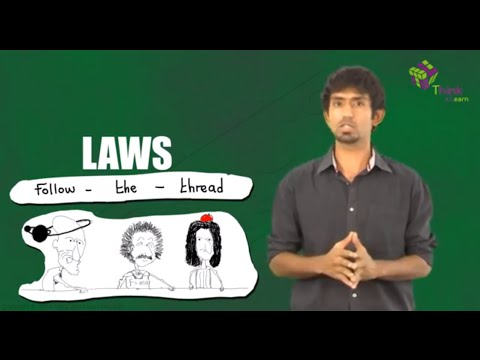## Laws of Motion Summary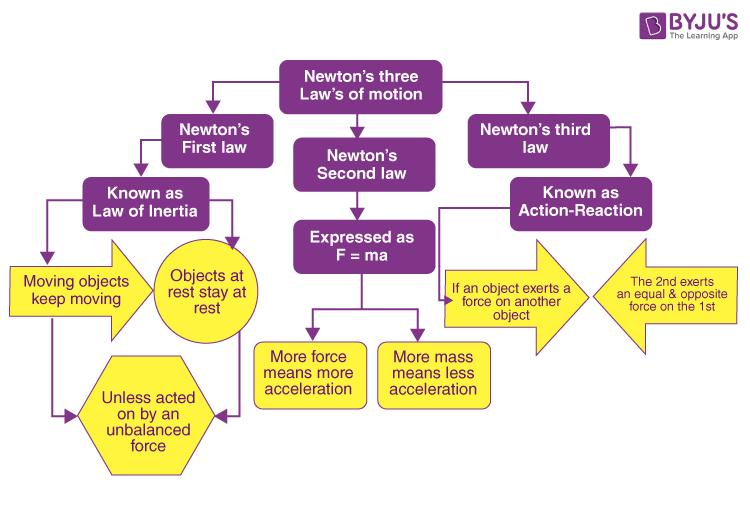## Laws of Motion Numericals

### 1. Suppose a bike with a rider on it having a total mass of 63 kg brakes and reduces its velocity from 8.5 m/s to 0 m/s in 3.0 seconds. What is the magnitude of the braking force?

Solution:

The combined mass of the rider and the bike = 63 kg
Initial Velocity = 8.5 m/s
Final Velocity = 0 m/s
The time in which the bike stops = 3 s

The net force acting on the body equals the rate of change of an object’s momentum.

$$\begin{array}{l}F=\frac{\Delta p}{\Delta t}\end{array}$$

The momentum of a body with mass m and velocity v is given by  p = mv

Hence, the change in momentum of the bike is given by

$$\begin{array}{l}\Delta p=mv-mu=m(v-u)\end{array}$$

Hence, the net force acting on the bike is given by

$$\begin{array}{l}F=\frac{m(v-u)}{\Delta t}\end{array}$$

Substituting the value, we get

$$\begin{array}{l}F=\frac{63\,kg\times (0-8.5\,m/s)}{3.0\,s}\end{array}$$
$$\begin{array}{l}F=-178.5\,N\end{array}$$

The magnitude of the braking force is -178.5 N.

### 2. Calculate the net force required to give an automobile of mass 1600 kg an acceleration of 4.5 m/s2.

We calculate the force using the following formula.

$$\begin{array}{l}F=ma\end{array}$$

Substituting the values in the equation, we get

$$\begin{array}{l}F=100\,kg\times 4.5\,m/s^2=7200\,N\end{array}$$
$$\begin{array}{l}F=7200\,N\end{array}$$

## Frequently Asked Questions – FAQs

### Who discovered the three laws of motion?

Sir Isaac Newton discovered the three laws of motion.

### Why are the laws of motion important?

Newton’s laws are essential because they relate to everything we do or see in everyday life. These laws tell us how things move or stay still and why we don’t float out of our bed or fall through the floor of our house.

### What are Newton’s laws of motion all about?

Newton’s laws of motion imply the relationship between an object’s motion and the forces acting on it. In the first law, we understand that an object will not change its motion unless a force acts on it. The second law states that the force on an object is equal to its mass times its acceleration. And finally, the third law states that there is an equal and opposite reaction for every action.

### What is the difference between Newton’s laws of motion and Kepler’s laws of motion?

Newton’s laws of motion are general and apply to any motion, while Kepler’s laws apply only to planetary motion in the solar system.

### What are some daily life examples of Newton’s 1st, 2nd and 3rd laws of motion?

• The motion of a ball falling through the atmosphere or a model rocket being launched up into the atmosphere are both excellent examples of Newton’s 1st law.
• Riding a bicycle is an excellent example of Newton’s 2nd law. In this example, the bicycle is the mass. The leg muscles pushing on the pedals of the bicycle is the force.
• You hit a wall with a certain amount of force, and the wall returns that same amount of force. This is an example of Newton’s 3rd law.

#### Session on Newton’s Laws of Motion.#### Top 10 Most Important and Expected Questions on Laws of Motion.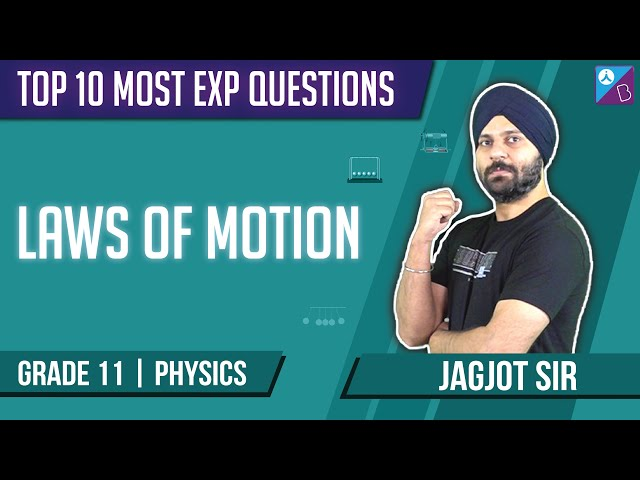Stay tuned to BYJU’S and Fall in Love with Learning!

Test your knowledge on Laws of motion

1. siddharth singh baghel

it is very helpful and useful i want to thank BY JU’S

2. Nouseen

Super

3. neha bhange

Good enough

• prathmesh shewale

nice

• prathmesh shewale

good

4. Thanks for the information

5. mohd mujeeb

Very good super

6. Kruthika. B

Excellent

7. Angela Sherpa

Good notes

9. Aishwarya

Good information!

10. Man suryawanshi

Nice exaplaination

The concepts are clear and easy to understand and to study. so, i feel comfortable with this app .

12. Good article

13. Pranjal Borkar

Good explanation of concept.

14. Good

15. Anantya

Thanks
This really helped me😀
I will secure 🥇🏆

16. Md Mohsin

Super Explanation

Good to learn

18. Shweta Singh

Thanks, BYJU’S.

19. Satyarth Khajuria

The best content for visualization and it cleared all my doubts. I personally think that BYJU’S is the best app in terms of e-learning. Thanks to BYJU’S

20. Monica

Neat and Crisp Content!

21. Vishwateja

Wow! Super and very useful content provided here.

22. Dhruvitha

A very useful content for making notes

Nice one

24. Thank you so much you helped in my project I ended up citing almost all my work from your website😅

25. Nice

26. It was nice 🙂

27. Anshika